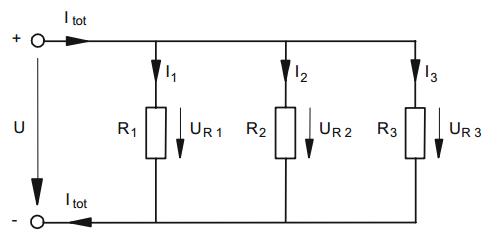Physics
Parallel circuiting of Resistors.
Author
Gintautas Stonys
Target Group
Student
Brief Description
Brief explanation of Parallel circuiting of Resistors

Share

parallel circuit is a closed circuit in which the current divides into two or more paths before recombining to complete the circuit. Each load connected in a separate path receives the full circuit voltage, and the total circuit current is equal to the sum of the individual branch currents.If resistors or quite generally loads are circuited in parallel they are all under the same voltage.

U = U R1 + U R2 + U R3

A partial current is flowing in every branch of a parallel circuit. The sum of all partial currents gives the total current.

Itot = I1 + I2 + I3